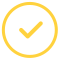effortlessly.

# What is Absolute Extrema?

It refers to the most extensive and smallest values of a function. For instance, the highest profit a company can make, the least quantity of cement needed to complete a building, and much more. Knowing how to find absolute extrema of a function is a daunting task to many students. In order to make the process easier for you, we've prepared this calculator - feel free to use it online.

## How To Use The Absolute Extrema Calculator

Finding the absolute extrema is very easy with our calculator. All you need to do is to:

• Identify the critical numbers, and
• Enter them into the function on the calculator

However, are you aware that there is a way to find absolute extrema without a calculator?

So first, let us look at some of the comparisons of absolute extrema.

### Local vs. Absolute Extrema

An absolute maximum occurs at the x value where the function is the biggest. In contrast, a local maximum occurs at an x value if the function is more prominent than points around it (i.e., an open interval around it).

A local minimum occurs at an x value if the function is smaller than the points around it. In contrast, an absolute minimum occurs at the x value where the function is the smallest (i.e., an open interval around it).

### Absolute vs. Relative Extrema

The absolute extrema will refer to the absolute minimums and maximums, while the relative extrema will refer to the relative minimums and maximums. Note that relative extrema do not occur at the endpoints of a domain. Unlike the absolute extrema, they only occur interior to the domain.

### Formula For Absolute Extrema Calculator With Interval

For absolute extrema, we should first have a continuous function, f(x), on an interval [a, b]. Therefore, using the function, we can be able to find the absolute extrema in the following steps:

• First, verify that the function is continuous
• Then find all the critical points of f(x) that are in the interval [a, b]
• Evaluate the function at the critical points derived from step 2 and the endpoints
• Finally, identify the absolute extrema

You can see that the procedure above does not take much professional writing help. Provided you have the critical points and evaluate them, finding the absolute extrema becomes easy-peasy.

Now, there are other ways of finding the absolute extrema:

### How To Find the Absolute Extrema of the Function on the Closed Interval Calculator

Using this method requires you to compute a derivative. Below is how to go about this process:

• Identify all critical numbers of f within the interval i.e. f'(x) = 0,
• Solve for x
• Consider only those solutions of x that satisfy a ≤ x ≤ b.
• Enter the values into the function f(x).
• Enter the endpoints, a and b, into the function f(x).

The immense value becomes the absolute maximum from the results you get, while the smallest value becomes the absolute minimum. You can use the absolute extrema calculator on interval to arrive at your answer.

### How To Find Absolute Extrema on a Graphing

Once you’ve found all the critical numbers of f within the interval [a, b], you can move on to plug the values on your graph paper. Draw the graph to arrive at your absolute minimum and maximum points.

Example:

Find the absolute extrema for:

g (t)=2t3+3t2−12t+4 on [−4,2]

Solution:

g′ (t) =6t2+6t−12=6(t+2) (t−1)

t = −2 and t = 1

Therefore:

g (-2) = 24

g (-4) = -28

g (1) = -3

g (2) = 8

### Pay Someone To Do My Math

Are you saying, “I want somebody to do my math homework for me?” We offer professional help with math homework at cheap rates.

Try our expert online service today!

Let’s make your assignment go away.Awesome. Now you'll never miss out.